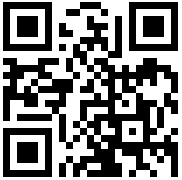4000-333-136

#### BIM培训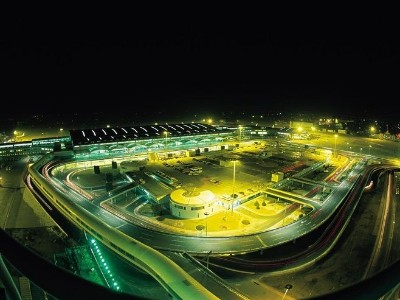### BIM应用创新杯获得大奖的项目，都是BIM标杆

2019-08-30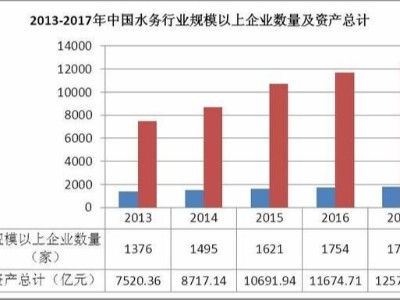### 中国水务行业发展存在的问题

1、人均水资源占有量少我国水资源总量为2.8万亿立方米，人均水资源量为2220立方米，预测到2030年人口增至15亿时，人均水资源量将降到1660立方米。按国际上一般承认的标准，我国为人均水资源少于1700立方米的用水紧张国家。有关专家发出警告，从2010年开始，我国就将进入严重缺水期，而到2030年，我国所有可利用的水资源都可能被“吃光用尽”。
2019-08-29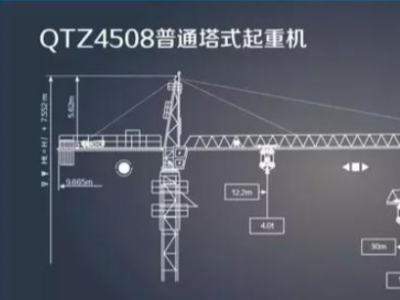### 塔吊施工与BIM应用

2019-08-29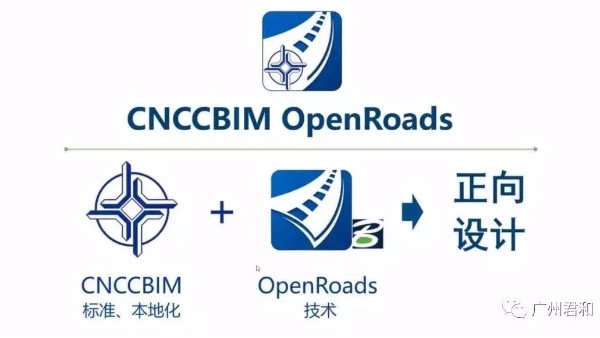### 公开课 | CNCCBIM Open Roads 教程

2020-03-09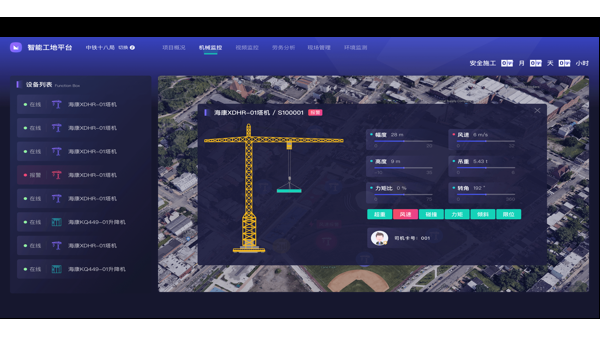### 智慧工地整体解决方案诚邀体验

2020-02-132019-12-03

# 建筑工程施工质量验收术语

01=

02=

03=04=

05=

06=

07=08=

09=

10

11

12

13

1401=

02=03=

04=

05=06=

07=

08=

09=

1001=

02=

03=

04=05=

06=

07=

08=

09=

1011

12

13

14

【相关阅读】
• 400电话

4000-333-136
• 微信扫一扫